Ex 4.3

Chapter 4 Class 9 Linear Equations in Two Variables
Serial order wise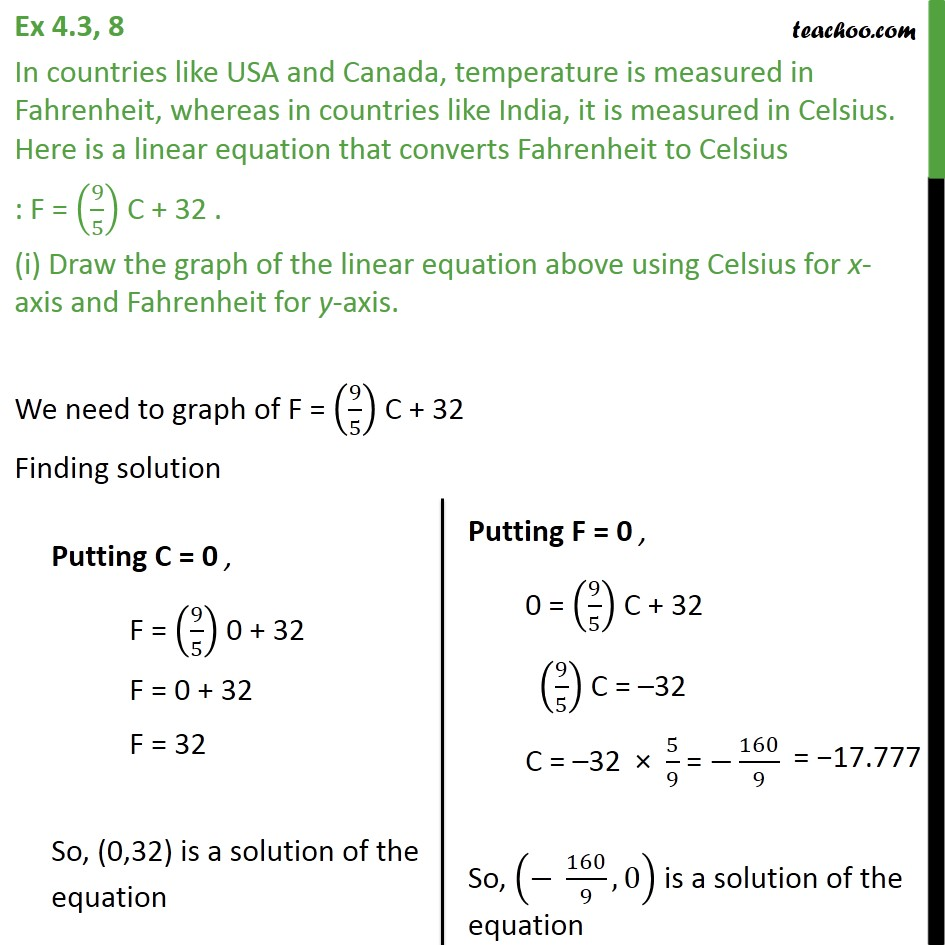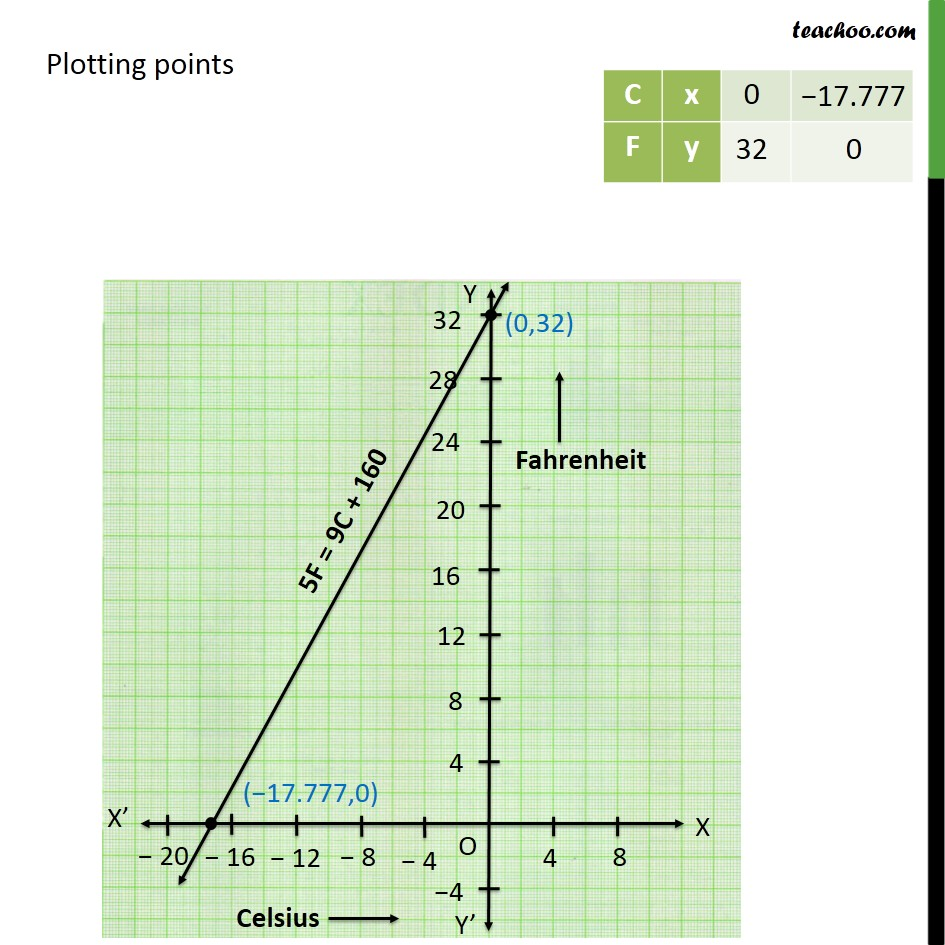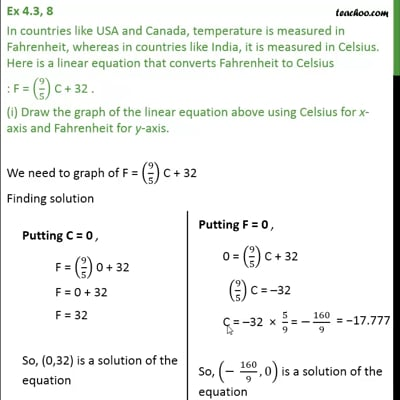This video is only available for Teachoo black users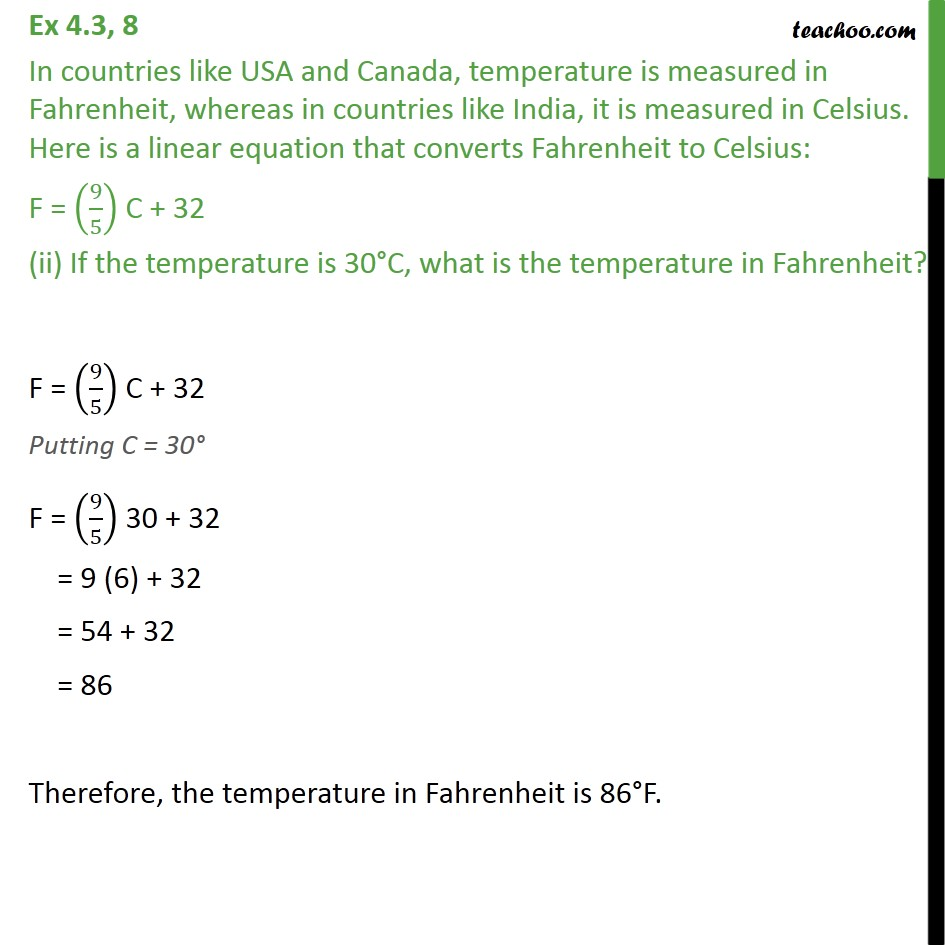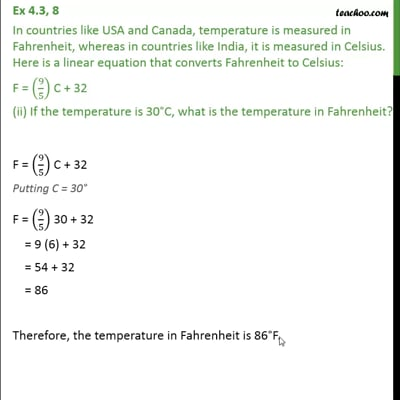This video is only available for Teachoo black users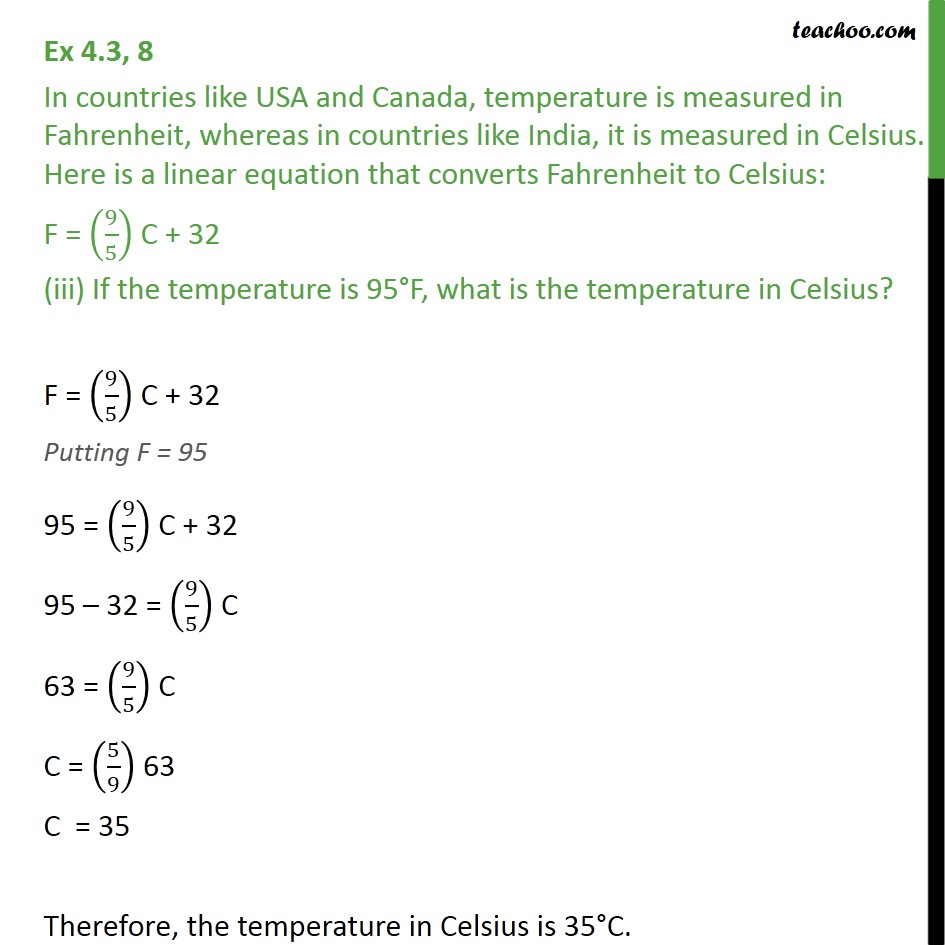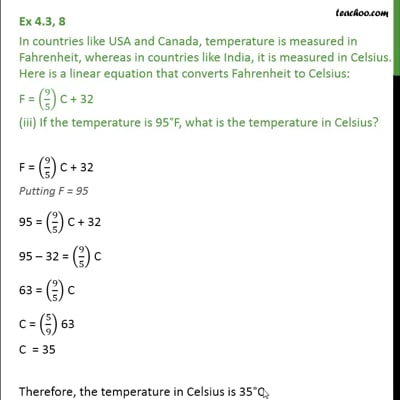This video is only available for Teachoo black users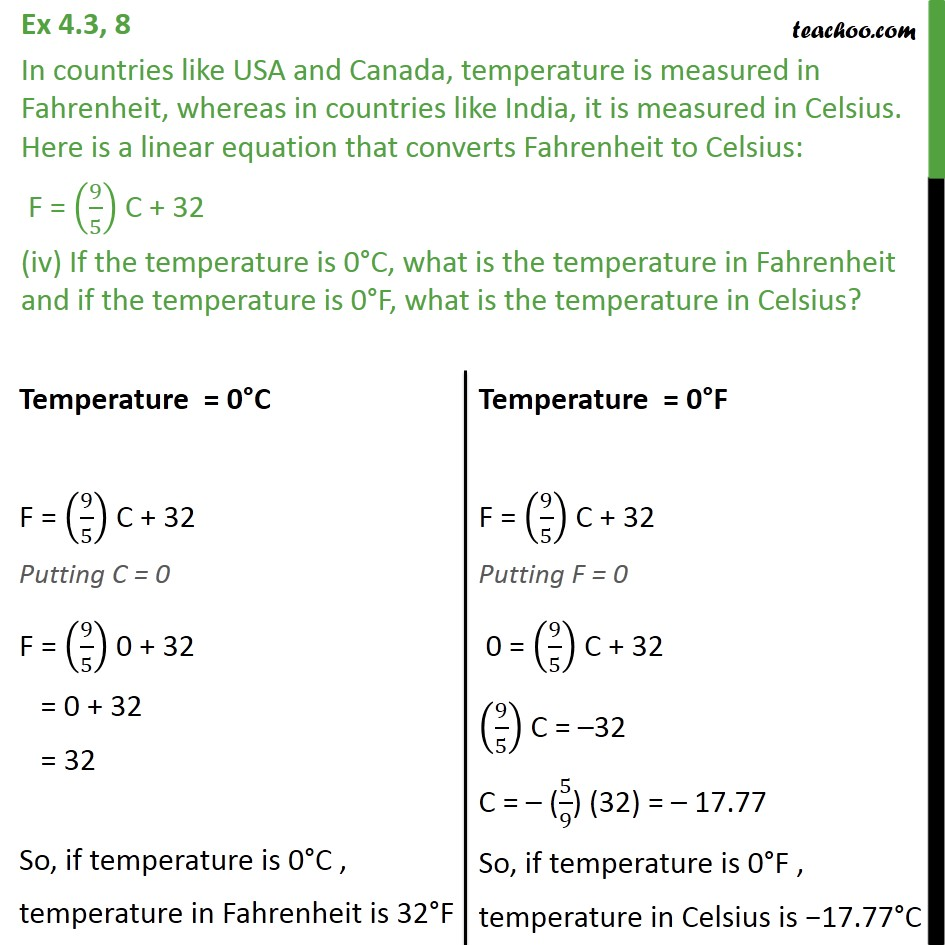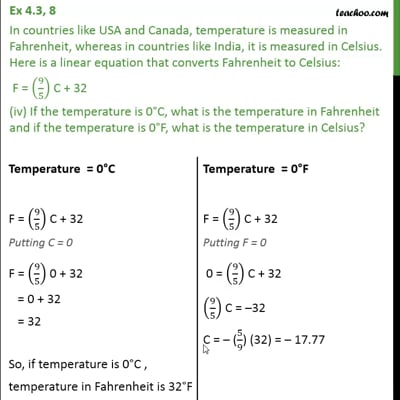This video is only available for Teachoo black users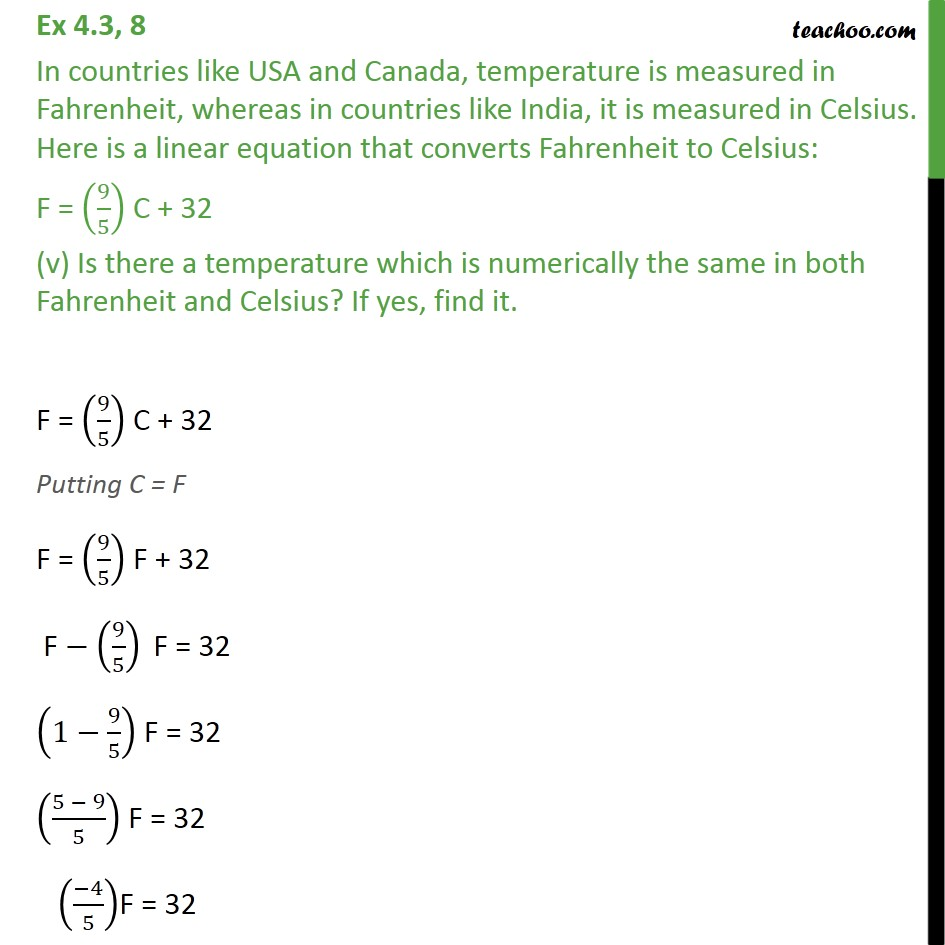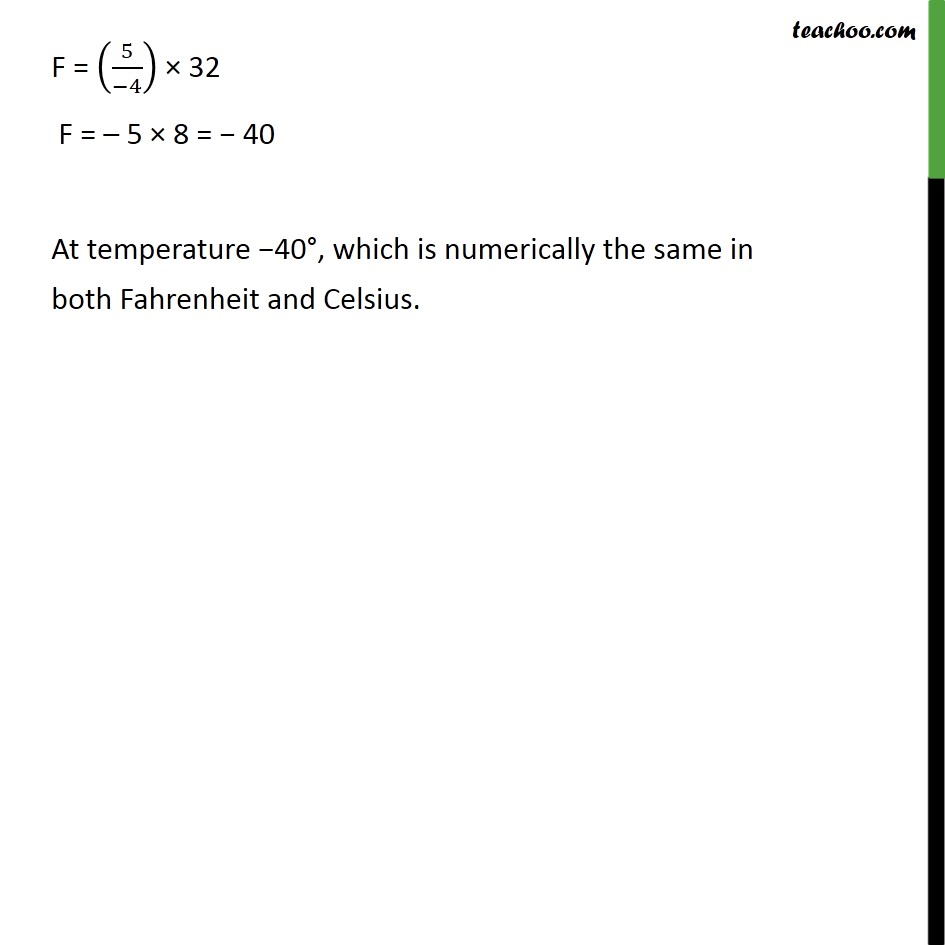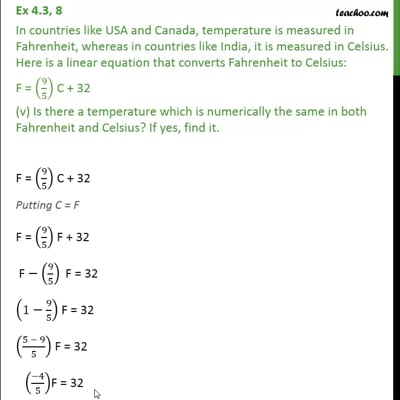This video is only available for Teachoo black users

Maths Crash Course - Live lectures + all videos + Real time Doubt solving!

### Transcript

Ex 4.3, 8 In countries like USA and Canada, temperature is measured in Fahrenheit, whereas in countries like India, it is measured in Celsius. Here is a linear equation that converts Fahrenheit to Celsius : F = (9/5) C + 32 . (i) Draw the graph of the linear equation above using Celsius for x-axis and Fahrenheit for y-axis. We need to graph of F = (9/5) C + 32 Finding solution Plotting points Ex 4.3, 8 In countries like USA and Canada, temperature is measured in Fahrenheit, whereas in countries like India, it is measured in Celsius. Here is a linear equation that converts Fahrenheit to Celsius: F = (9/5) C + 32 (ii) If the temperature is 30 C, what is the temperature in Fahrenheit? F = (9/5) C + 32 Putting C = 30 F = (9/5) 30 + 32 = 9 (6) + 32 = 54 + 32 = 86 Therefore, the temperature in Fahrenheit is 86 F. Ex 4.3, 8 In countries like USA and Canada, temperature is measured in Fahrenheit, whereas in countries like India, it is measured in Celsius. Here is a linear equation that converts Fahrenheit to Celsius: F = (9/5) C + 32 (iii) If the temperature is 95 F, what is the temperature in Celsius? F = (9/5) C + 32 Putting F = 95 95 = (9/5) C + 32 95 32 = (9/5) C 63 = (9/5) C C = (5/9) 63 C = 35 Therefore, the temperature in Celsius is 35 C. Ex 4.3, 8 In countries like USA and Canada, temperature is measured in Fahrenheit, whereas in countries like India, it is measured in Celsius. Here is a linear equation that converts Fahrenheit to Celsius: F = (9/5) C + 32 (iv) If the temperature is 0 C, what is the temperature in Fahrenheit and if the temperature is 0 F, what is the temperature in Celsius? Ex 4.3, 8 In countries like USA and Canada, temperature is measured in Fahrenheit, whereas in countries like India, it is measured in Celsius. Here is a linear equation that converts Fahrenheit to Celsius: F = (9/5) C + 32 (v) Is there a temperature which is numerically the same in both Fahrenheit and Celsius? If yes, find it. F = (9/5) C + 32 Putting C = F F = (9/5) F + 32 "F" (9/5)" F "= 32 (1 9/5) F = 32 ((5 9)/5) F = 32 (( 4)/5)F = 32 F = (5/( 4)) 32 F = 5 8 = 40 At temperature 40 , which is numerically the same in both Fahrenheit and Celsius.